# Adding and Subtracting Fractions with Like Denominators

Imagine that you and a friend are eating part of a pizza with 8 slices.

You have eaten 3 of the 8 pieces and your friend has eater 2 of the 8 pieces.We can represent the pizza you at asThe pizza your friend at is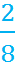of the pizza.

So how much of the pizza did the both of you eat? You are 5 of the 8 pizzas, so that makes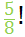Let's look at what happened mathematically with the fractions.So, when we add fractions with the same denominator, or like denominators, we add the numerator and leave the denominator alone.

Here are a couple more examples:

Example #1: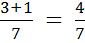=Example #2:You can take this example another step because 8 and 16 have a common factor.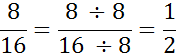Example #3:The final answer in this example is an improper fraction. So you could change it to an improper fraction.Now imagine that you go to that pizza box and there are 7 pieces left. We will call thisof a pizza. You eat 4 pieces, orof the pizza. What fractional amount of the pizza is left?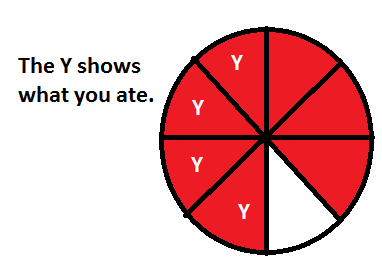There are 3 pieces left, orof the pizza is left. Let's look again at the math behind the scenes.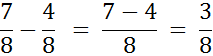So we subtract the numerators and leave the denominators alone.

Check out a few more examples.

Example #4:Example #5: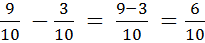This answer can be reduced!Let's Review:

If you have common denominators, you can just add the numerators or just subtract the numerators. Be sure to leave the denominator the same. Sometimes you might want to reduce, or simplify your answer. Divide by a common factor to simplify the fraction.

Math
Fractions